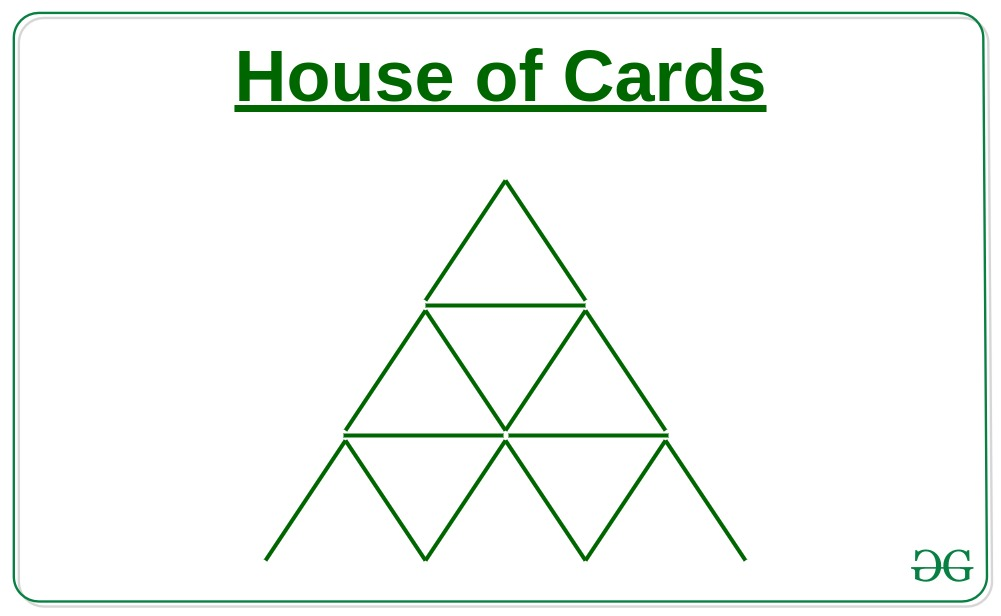# Number of cards needed build a House of Cards of a given level N

• Difficulty Level : Easy
• Last Updated : 21 Dec, 2021

Given a number N, the task is to find the number of cards needed to make a House of Cards of N levels.Examples:

Input: N = 3
Output: 15
From the above image, it is clear that for the House of Cards for 3 levels 15 cards are needed
Input: N = 2
Output:

Approach:

1. If we observe carefully, then a series will be formed as shown below in which i-th item denotes the number of triangular cards needed to make a pyramid of i levels:

2, 7, 15, 26, 40, 57, 77, 100, 126, 155………and so on.

1.
2. The above series is a method of difference series where differences are in AP as 5, 8, 11, 14……. and so on.

3. Therefore nth term of the series will be:

```nth term = 2 + {5 + 8 + 11 +14 +.....(n-1) terms}
= 2 + (n-1)*(2*5+(n-1-1)*3)/2
= 2 + (n-1)*(10+(n-2)*3)/2
= 2 + (n-1)*(10+3n-6)/2
= 2 + (n-1)*(3n+4)/2
= n*(3*n+1)/2;```
1.
2. Therefore the number of cards needed for building a House of Cards of N levels will be:

``
1.

Below is the implementation of the above approach:

## CPP

 `// C++ implementation of the above approach` `#include ``using` `namespace` `std;` `// Function to find number of cards needed``int` `noOfCards(``int` `n)``{``    ``return` `n * (3 * n + 1) / 2;``}` `// Driver Code``int` `main()``{``    ``int` `n = 3;``    ``cout << noOfCards(n) << ``", "``;``    ``return` `0;``}`

## Java

 `// Java implementation of the above approach``import` `java.lang.*;` `class` `GFG``{``    ``// Function to find number of cards needed``    ``public` `static` `int` `noOfCards(``int` `n)``    ``{``        ``return` `n * (``3` `* n + ``1``) / ``2``;``    ``}``    ` `    ``// Driver Code``    ``public` `static` `void` `main(String args[])``    ``{``        ``int` `n = ``3``;``        ``System.out.print(noOfCards(n));``    ``}``}` `// This code is contributed by shubhamsingh10`

## Python3

 `# Python3 implementation of the above approach` `# Function to find number of cards needed``def` `noOfCards(n):``    ``return` `n ``*` `(``3` `*` `n ``+` `1``) ``/``/` `2` `# Driver Code``n ``=` `3``print``(noOfCards(n))` `# This code is contributed by mohit kumar 29`

## C#

 `// C# implementation of the above approach``using` `System;` `class` `GFG``{``    ``// Function to find number of cards needed``    ``public` `static` `int` `noOfCards(``int` `n)``    ``{``        ``return` `n * (3 * n + 1) / 2;``    ``}``     ` `    ``// Driver Code``    ``public` `static` `void` `Main(String []args)``    ``{``        ``int` `n = 3;``        ``Console.Write(noOfCards(n));``    ``}``}` `// This code is contributed by 29AjayKumar`

## Javascript

 ``

Output:

`15`

Time Complexity: O(1)

Auxiliary Space: O(1)

My Personal Notes arrow_drop_up